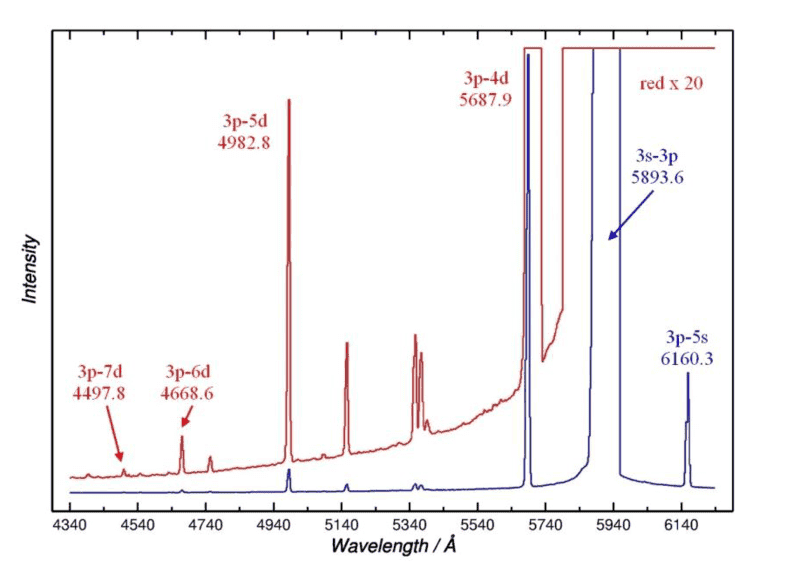# Calculating Quantum Defect for Na I 3p-nd, n=4-7 Terms

• Philip Land
In summary, the spectrum shows the series 3p - nd, n = 4 - 7 in Na as well as the resonance line 3s - 3p, with the experimental vacuum wavelengths in Å. The equation for calculating the quantum defect for nd terms is T = R\frac{(z-N_{inner})^2}{(n-\delta)^2}. However, due to the high delta values, the calculated defect may be inaccurate.

## Homework Statement

The spectrum shows the series 3p - nd, n = 4 - 7 in Na as well as the resonance line 3s - 3p, with the experimental vacuum wavelengths in Å.

Calculate the quantum defect for the nd ##^2D## n = 4-7 terms. Estimate, as accurately as possible, the wavelength for 3p - 8d. The ionization energy in Na I is 41449.6 cm-1. Neglect all finestructure.

## Homework Equations

##E_{ionization} - E_{excitation} = T = R\frac{(z-N_{inner})^2}{(n-\delta)^2}##

## The Attempt at a Solution

Hi!

I can take the inverse of the given wavelength, to get T. ##\frac{1}{\lambda} = T##.

Then I can plug this into the above equation and solve for delta. ##\delta = n - sqrt(\frac{R*(z-N_{inner})^2)}{T})##

But my question is. Do I also need to take the quantum defect for p into account?

Where my ##T = R*(z-N_{inner})^2 ( \frac{1}{(n-\delta_p)^2} - \frac{1}{(n-\delta_d)^2}) ##

And if so, how would I get ##\delta_p##?

If it was s, I could impy solve for ##delta_s## when putting ##T= E_{io}##.

Figure attached#### Attachments

Philip Land said:
But my question is. Do I also need to take the quantum defect for p into account?
From the data you have, can't you calculate E(3p) and then find the quantum defect from energy levels relative to that?

•Philip Land
DrClaude said:
From the data you have, can't you calculate E(3p) and then find the quantum defect from energy levels relative to that?

Thanks. I actually think I can by looking at the blue lines. I can put 6140 = ##R( \frac{1}{(5- \delta_s)^2}-\frac{1}{(3-\delta_p)^2})## Where quantum defect for s is ##\delta_s = 3- sqrt( \frac{R}{E_{io}}.## I guess.

On the right track? :)

There's no facit so I can't really tell if the asnswer is correct.

DrClaude said:
From the data you have, can't you calculate E(3p) and then find the quantum defect from energy levels relative to that?
But doing this i get

delta p = 2.739

delta s = 1.373

delta d = 3.1356

Which seems to be to high values.